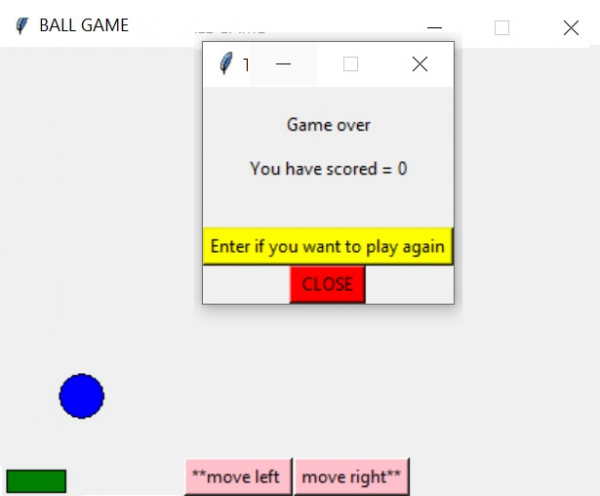# Catching the ball game using Python

Python can also be used to create computer games. In this article we will see how the ball catching game can be created using python. In this game a ball keeps falling from the top of a canvas window and a bar is present at the bottom of the window. Two buttons are provided to move the bar in the left and right direction. Using mouse button presss we move the bar at the bottom to catch the falling ball. At different times the ball falls from different positions .

## Approach

The approach to build the game is described in the following steps.

• Step  1  − Draw a tkinter rectangular canvas that can be used to hold further layouts like various graphics, text and images etc.

• Step 2 − Create the ball that is going to fall from the top. Use the create_oval method for it. It takes 4 coordinates to create a oval which is a mixture of circle and rectangle.

• Step  3 − Create the bar at the bottom that will move from left to right on mouse button press. The create_rectangle method can be used for this.

•  Step 4 − Use the canvas.move method to move the ball or bar. This method is used for both vertical and horizontal movement of the objects it is attached to.

• Step 5  − Create the buttons that will be used for moving the bar at the bottom. There will be events applied to it which will trigger when the buttons are clicked.

## The Program

Below is the complete program that is based on the above steps using the relevant methodsand objects.

## Example

#Catching the ball game using Python

from tkinter import Tk, Button, Label
from tkinter import Canvas
from random import randint
base = Tk()
base.title("BALL GAME")
base.resizable(False, False)
color = Canvas(base, width=590, height=610)
color.pack()
standard = 0
length = 5
marks = 0
class model:
def __init__(self, color, m1, n1, m2, n2):
self.m1 = m1
self.n1 = n1
self.m2 = m2
self.n2 = n2
self.color = color
self.circle = color.create_oval(self.m1, self.n1, self.m2, self.n2,fill="blue", tags='dot1')
def Game(self):
offset = 5
global standard
if standard >= 510:
global length, marks, next
if (length - offset <= self.m1 and
length + 40 + offset >= self.m2):
marks += 5
color.delete('dot1')
game_play()
else:
color.delete('dot1')
slide.remove(self)
result()
return

standard += 1
self.color.move(self.circle, 0, 1)
self.color.after(10, self.Game)
class slide:
def __init__(self, color, m1, n1, m2, n2):
self.m1 = m1
self.n1 = n1
self.m2 = m2
self.n2 = n2
self.color = color
self.num = color.create_rectangle(self.m1, self.n1, self.m2, self.n2, fill="green",       tags='dot2')
def push(self, num):
global length
if (num == 1):
self.color.move(self.num, 20, 0)
length += 20
else:
self.color.move(self.num, -20, 0)
length -= 20
def remove(self):
color.delete('dot2')
def game_play():
global standard
standard = 0
size = randint(0, 570)
game1 = model(color, size, 20, size + 30, 50)
game1.Game()
def result():
base2 = Tk()
base2.title("THE BALL GAME")
base2.resizable(False, False)
set = Canvas(base2, width=300, height=300)
set.pack()
z = Label(set, text="\nGame over\n\nYou have scored = " + str(marks) + "\n\n")
z.pack()
btx = Button(set, text="Enter if you want to play again", bg="yellow", command=lambda:    repeat(base2))
btx.pack()
bty = Button(set, text=" CLOSE ", bg="red",command=lambda: destroy(base2))
bty.pack()
def repeat(base2):
base2.destroy()
function()
def destroy(base2):
base2.destroy()
base.destroy()
def function():
global marks, length
marks = 0
length = 0
x1 = slide(color, 5, 560, 45, 575)
Bt0 = Button(color, text="move right**", bg="pink",command=lambda: x1.push(1))
Bt0.place(x=335, y=580)
Bt1 = Button(color, text="**move left ", bg="pink", command=lambda: x1.push(0))
Bt1.place(x=260, y=580)
game_play()
base.mainloop()
if (__name__ == "__main__"):
function()

## Output

Running the above code gives us the following result −